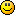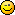# Lets do a little math

1. Okay what is your favorite mathematical equation or solution.  This is a fun way for everyone to learn mathematics.

Do you ever wonder how to locate the vertex on a parabola?

Oh how I wish I could draw.Three ways to find the vertex for a parabola y=x(squared) +10x +21

X intercept form.
This eqantion is factored to (x+7)(x+3)=y and will have zeros (where the function crosses the x axis) at -3 and -7.  The vertex's x value will be half way between -3 and -7 or -5.  If this is the case, it will have a y value of (-5+7)(-5+3)=-4  The vertex will be at (-5,-4).

Completing the square:

To complete the square, we take 1/2 of the B term and have this equation...........(x+5)squared.  We then subtract 25 or 1/2 of b squared from 21 and we have (x+5) squared -4=y.  This is of the form (x-h) squared +k=y.  -h=5 and h=5 k=-4.
Voila, the vertex is (-5,-4)

Take the derivative to find the min (or max)if the parabola opens downward.  A parabola will only have one min or max so it doesnt matter testing for which one it is.  Okay first we take the derivative of the equation x(squared)+10x+21=y.  dy/dx= 2x+10=0 so x=-5 and if we plug back into the original equation we will get the vertex at (-5,-4).

Pretty nifty huh?  Okay I heard the snickering and nerd shouted in the background.

I would like to see some mathematical methods that others want to share whether it is factoring prime numbers or solutions to green's function.

1.0

Let us define our differential equation of the form:

M(x,y)dx + N(x,y)dy = 0

If M(x,y) and N(x,y) are of the same degree the equation can be said to be homogeneous.  The degree of an equation is defined by the highest power of the variables.

ie. x^2 +2x + 1 is an equation of order 2
x^2 + xy + y^2 is also an equation of order 2

This can be illustrated another way.  A differential equation is homogeneous if the following is true:

M(tx,ty) = (t^n)M(x,y) and N(tx,ty) = (t^n)N(x,y)

To solve an homogeneous differential equation one can use algebraic substitution with either y = ux or x = vy.  Effectively this reduces the order of the differential equation to a separable first-order differential equation as seen below:

y = ux
dy = udx + xdu

M(x,ux)dx + N(x,ux)[udx+xdu] = 0

Upon simplification this equation becomes easy to solve using the basic principles of seperable variables.  I will illustrate with an example:

(x^2 + y^2)du + (x^2 - xy)dy = 0
(x^2 + (u^2)(x^2))dx + (x^2 - ux^2)[udx + xdu] = 0
(x^2)(1+u)dx + (x^3)(1-u)du = 0
1-u       dx
---- du + -- = 0
1+u        x
(-1 + 2/(1+u))du + (dx/x) = 0

Now you can take the integral of Mdu and Ndx to get:

-u + 2ln|1+u| + ln|x| = ln|c|

Resubstitute u=y/x:

(-y/x) + 2ln|1+ (y/x)| + ln|x| = ln|c|
ln|((x+y)^2)/(cx)| = y/x

The final solution being:

(x+y)^2 = cxe^(y/x)

This is arrived it using the definition of a logarithm:

a^b = c is defined as as loga(c) = b

And loge = ln

1. Homogeneous deq's are great.  I like first order differential equations that are used to solve equations for RC or RL

Thank you, that was nice.

1.0

I love the partial DEs we use with the heat and vorticity equations!

2. Instead of doing something complex like using Maxwell's equations to describe magnetic fields lets factor a number to its prime roots.  How do we do this?

Lets look at 24.

We first divide by 2.
So we have 24
2   12

We then see that 12 can be divided by 2 again (actually twice).

so we have 24
2   12
2    6
2   3

The prime roots of 24 are 2,2,2, & 3

So what we actually do is to divide by the first prime number (2) until the number cannot be divided (to produce and integer result) by that number any more.   We keep dividing by higher and higher prime numbers until  all the factors are prime.

Okay lets take 5460...............For brevity, I am going to skip some steps.  Following is the result:

5460
2   2730
2   1365
3   455
5   91
7  13

The prime factors of 5460 are 2, 2, 3, 5, 7, & 13.

Now you can use this to reduce fractions and find things like "least common multiple".  We will talk about that later.2. I'm not a "big Math expert", so I'm pretty limited in my selections as far as any favorites go; but I absolutely love The Mandelbrot Set (multiply Z by itself and add C" to get a "new Z".

Fractals can show how it works in action; but if someone understands the concept he'll see it at work in Nature (and even in something like society) everywhere.

http://www.cygnus-software.com/theory/theory.htm

1.0

Ooo, that is most definitely another fantastic one!

3. Dirac's equation (relativistic Schrodinger's equation).

1. Dirac's equation is quite a nice work.  While I am not an expert in this area, quantum mechanics & relativity require quite interesting math.

I wish I knew a little more in this area.

Now that has some pretty tricky derivatives.......both in math and in options.  Okay that was a bad joke.

4. One of my faves:

.999~ = 1

x = .999~
so 10x = 9.999~
9.999~ - .999~ = 9
but 10x - x = 9x
so 9x = 9, x = 1
Thus, x = .999~ = 11. That was so, elementary...  It took all that hogwash to say the infinitive decimals within ".999" basically equals 1?  Ha-ha!  I've got one even better:

X = 1 moronic mathematician

3x + 2x = 5 crazed lunatics

Yikes!

LOL!1. Unfortunately your equation should read 5x=5 moronic mathematicians.  If you are letting x=1 moronic mathematician, you must stay in convention.
However, if you let 1 moronic mathematician =1 crazed lunatic, you would be right.)

Very clever.

Thanks for your input1. That was my point:  1 moronic mathematician equals 1 crazed lunatic.
I'm glad you understood the jest of my math joke.

Thanks for your calculations.1. You are very welcome. What are friends for?  I was wondering if you could teach me sarcasm?  You seem to be quite good at it.

I hear that sarcasm is a cure for insanity and some mild cases of retardation which most mathemeticians exhibit.

1. Your last sentence:  Was that a sarcastic claim?  Ha-ha!
Sarcasm does, at times, bring light to the dark madness.1. Oh maybe I did make a sarcasm.

I think I am getting over my agoraphobia.

Okay I will stay inside.  I promise.

1. You must be getting over your agoraphobia condition, since you're live via cyber-hogwash.  It takes time, dear hermit; work your way outside in a slow fashion and apply sunscreen, since it has been awhile.  Ha-ha!

1. That sounds like good advice.  It looks like both of us are posting a lot of garbage.  Oh yes that is how you came up with cyber-hogwash.  What I would like to do is to become cyber-sociopath like you.

Here is the thing.  You were able to bait me into a conversation and readytoescape couldnt get to reply.  You do have a talent you know.

I do have some sunscreen.  The light is going to be bit bright yes-sir-ree.  Thank you for your kind advice.

5. Social math: 1 + 1 eventually equals 3 or 4 or more

6. Academia + Humor typically equals a silent house

1. Apparently, I have been mathematically proven correct.

16 minutes & what ….crickets

7. Theorem: a cat has nine tails.

Proof:
No cat has eight tails.
A cat has one tail more than no cat.
Therefore, a cat has nine tails.

1. Thank you Disturbia.

Logic is very interesting.  It is like Escher painting with words1. You're very welcome Ben.  I spent a long time pondering that 9-tail cat theorem... LOL!

I studied art in college, among other things... I'm very familiar with the works of M.C. Escher.  I will take any comparison to him on any level as a complement.

working